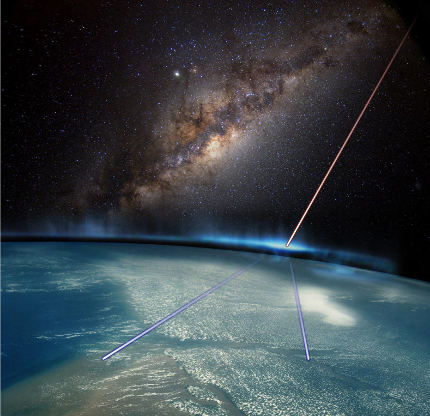##### Page tree
Go to start of banner

# Technique

## Cosmic Rays

Every second, a shower of muons flows through the atmosphere, crawls through our bodies and through everything it crosses.
Those particle are originated in the stratospheric region of the Earth from the interaction of Cosmic Rays with the highest layers of the atmosphere. Cosmic Rays are mainly composed of H+ and He++  (p and α particles) generated outside out solar system, in the inner regions of our galaxy or coming from extragalactic sources.

## Tomography Using Muons

Materials with high atomic number (Z) are able to bend the muons trajectory. This phenomenon, known as Coulomb scattering, can be used to determine a relative density three-dimensional map of the volume enclosed by a set of muon trackers. You can learn all the technical details reading the scientific literature on the argument.
If you just want a brief, simplified introduction, read on!

## A Step Inside the Physics

Since the behaviour of the scattering of heavy, charged particle inside a defined volume of matter is known, one can evince the distribution of the matter inside a volume given a defined measure of the scattering of such particles inside the said volume. In order to quantify this knowledge, we must bind the round mean squared of the distribution of the scattering angles θi of a set of particles crossing a distance Li to the radiation lenght X0 of the material as:where pi is the particle momentum and b=13.6MeV/c. The scattering density λ=1/X0 is proportional to the mass density ρ and the atomic number Z of the crossed material as follows:As a consequence, the ability to reconstruct a 3D map of the scattering density values of a closed volume under inspection allows to identify high Z, high ρ materials. This can be achieved measuring a large set of muons crossing the volume and scattering inside the enclosed matter. Given this dataset, it is possible to write the probability to have the 3D density distribution λ inside the volume as:where the index i runs over the muons of the dataset of size M. All the probabilities are considered Gaussian and the muon events uncorrelated. Using a maximization procedure it is possible to maximize this probability, obtaining the configuration of densities inside the volume that maximize the probability to observe the acquired data. We use an iterative MLEM algorithm to achieve this configuration. You can take a look at some 3D rendering of the reconstructed density map in the test cases section.A cosmic ray interacting with the upper atmosphere

• No labels